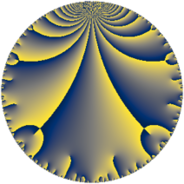# Properties

 Label 147.6.aLevel $147$ Weight $6$ Character orbit 147.a Rep. character $\chi_{147}(1,\cdot)$ Character field $\Q$ Dimension $35$ Newform subspaces $15$ Sturm bound $112$ Trace bound $4$

# Related objects

## Defining parameters

 Level: $$N$$ $$=$$ $$147 = 3 \cdot 7^{2}$$ Weight: $$k$$ $$=$$ $$6$$ Character orbit: $$[\chi]$$ $$=$$ 147.a (trivial) Character field: $$\Q$$ Newform subspaces: $$15$$ Sturm bound: $$112$$ Trace bound: $$4$$ Distinguishing $$T_p$$: $$2$$, $$5$$

## Dimensions

The following table gives the dimensions of various subspaces of $$M_{6}(\Gamma_0(147))$$.

Total New Old
Modular forms 102 35 67
Cusp forms 86 35 51
Eisenstein series 16 0 16

The following table gives the dimensions of the cuspidal new subspaces with specified eigenvalues for the Atkin-Lehner operators and the Fricke involution.

$$3$$$$7$$FrickeDim.
$$+$$$$+$$$$+$$$$9$$
$$+$$$$-$$$$-$$$$9$$
$$-$$$$+$$$$-$$$$10$$
$$-$$$$-$$$$+$$$$7$$
Plus space$$+$$$$16$$
Minus space$$-$$$$19$$

## Trace form

 $$35 q - 2 q^{2} - 9 q^{3} + 624 q^{4} - 38 q^{5} - 126 q^{6} - 552 q^{8} + 2835 q^{9} + O(q^{10})$$ $$35 q - 2 q^{2} - 9 q^{3} + 624 q^{4} - 38 q^{5} - 126 q^{6} - 552 q^{8} + 2835 q^{9} + 1128 q^{10} + 920 q^{11} - 828 q^{12} - 550 q^{13} + 450 q^{15} + 11196 q^{16} + 2286 q^{17} - 162 q^{18} - 3844 q^{19} + 6476 q^{20} - 4820 q^{22} - 1096 q^{23} - 2052 q^{24} + 14429 q^{25} - 2128 q^{26} - 729 q^{27} + 1174 q^{29} + 5292 q^{30} - 12016 q^{31} - 22776 q^{32} + 4716 q^{33} + 17664 q^{34} + 50544 q^{36} + 36032 q^{37} + 12296 q^{38} + 2952 q^{39} + 40236 q^{40} - 11002 q^{41} - 6394 q^{43} - 38272 q^{44} - 3078 q^{45} + 30584 q^{46} + 29856 q^{47} + 2304 q^{48} - 23086 q^{50} + 10386 q^{51} - 37660 q^{52} + 61814 q^{53} - 10206 q^{54} - 37944 q^{55} - 24246 q^{57} - 157816 q^{58} + 11508 q^{59} + 58140 q^{60} - 47590 q^{61} - 39264 q^{62} + 195740 q^{64} - 8448 q^{65} - 68112 q^{66} - 60726 q^{67} - 26820 q^{68} + 32400 q^{69} - 100324 q^{71} - 44712 q^{72} - 60706 q^{73} - 303640 q^{74} - 87687 q^{75} + 93008 q^{76} + 139968 q^{78} - 23594 q^{79} + 348548 q^{80} + 229635 q^{81} + 69648 q^{82} - 75540 q^{83} - 256044 q^{85} + 748444 q^{86} - 224622 q^{87} + 387924 q^{88} + 110710 q^{89} + 91368 q^{90} - 80024 q^{92} - 199458 q^{93} - 138240 q^{94} - 388652 q^{95} - 20340 q^{96} + 230150 q^{97} + 74520 q^{99} + O(q^{100})$$

## Decomposition of $$S_{6}^{\mathrm{new}}(\Gamma_0(147))$$ into newform subspaces

Label Dim $A$ Field CM Traces A-L signs $q$-expansion
$a_{2}$ $a_{3}$ $a_{5}$ $a_{7}$ 3 7
147.6.a.a $1$ $23.576$ $$\Q$$ None $$-6$$ $$-9$$ $$-6$$ $$0$$ $+$ $-$ $$q-6q^{2}-9q^{3}+4q^{4}-6q^{5}+54q^{6}+\cdots$$
147.6.a.b $1$ $23.576$ $$\Q$$ None $$-6$$ $$9$$ $$-78$$ $$0$$ $-$ $-$ $$q-6q^{2}+9q^{3}+4q^{4}-78q^{5}-54q^{6}+\cdots$$
147.6.a.c $1$ $23.576$ $$\Q$$ None $$-2$$ $$-9$$ $$11$$ $$0$$ $+$ $+$ $$q-2q^{2}-9q^{3}-28q^{4}+11q^{5}+18q^{6}+\cdots$$
147.6.a.d $1$ $23.576$ $$\Q$$ None $$-2$$ $$9$$ $$-11$$ $$0$$ $-$ $-$ $$q-2q^{2}+9q^{3}-28q^{4}-11q^{5}-18q^{6}+\cdots$$
147.6.a.e $1$ $23.576$ $$\Q$$ None $$1$$ $$9$$ $$34$$ $$0$$ $-$ $-$ $$q+q^{2}+9q^{3}-31q^{4}+34q^{5}+9q^{6}+\cdots$$
147.6.a.f $1$ $23.576$ $$\Q$$ None $$5$$ $$-9$$ $$-94$$ $$0$$ $+$ $-$ $$q+5q^{2}-9q^{3}-7q^{4}-94q^{5}-45q^{6}+\cdots$$
147.6.a.g $1$ $23.576$ $$\Q$$ None $$10$$ $$-9$$ $$106$$ $$0$$ $+$ $-$ $$q+10q^{2}-9q^{3}+68q^{4}+106q^{5}+\cdots$$
147.6.a.h $2$ $23.576$ $$\Q(\sqrt{193})$$ None $$-3$$ $$-18$$ $$72$$ $$0$$ $+$ $-$ $$q+(-1-\beta )q^{2}-9q^{3}+(17+3\beta )q^{4}+\cdots$$
147.6.a.i $2$ $23.576$ $$\Q(\sqrt{249})$$ None $$-3$$ $$-18$$ $$-33$$ $$0$$ $+$ $+$ $$q+(-1-\beta )q^{2}-9q^{3}+(31+3\beta )q^{4}+\cdots$$
147.6.a.j $2$ $23.576$ $$\Q(\sqrt{193})$$ None $$-3$$ $$18$$ $$-72$$ $$0$$ $-$ $-$ $$q+(-1-\beta )q^{2}+9q^{3}+(17+3\beta )q^{4}+\cdots$$
147.6.a.k $2$ $23.576$ $$\Q(\sqrt{249})$$ None $$-3$$ $$18$$ $$33$$ $$0$$ $-$ $-$ $$q+(-1-\beta )q^{2}+9q^{3}+(31+3\beta )q^{4}+\cdots$$
147.6.a.l $4$ $23.576$ $$\mathbb{Q}[x]/(x^{4} - \cdots)$$ None $$3$$ $$-36$$ $$0$$ $$0$$ $+$ $-$ $$q+(1-\beta _{1})q^{2}-9q^{3}+(18-\beta _{1}+\beta _{2}+\cdots)q^{4}+\cdots$$
147.6.a.m $4$ $23.576$ $$\mathbb{Q}[x]/(x^{4} - \cdots)$$ None $$3$$ $$36$$ $$0$$ $$0$$ $-$ $+$ $$q+(1-\beta _{1})q^{2}+9q^{3}+(18-\beta _{1}+\beta _{2}+\cdots)q^{4}+\cdots$$
147.6.a.n $6$ $23.576$ $$\mathbb{Q}[x]/(x^{6} - \cdots)$$ None $$2$$ $$-54$$ $$-100$$ $$0$$ $+$ $+$ $$q-\beta _{2}q^{2}-9q^{3}+(5^{2}-\beta _{1}+\beta _{4})q^{4}+\cdots$$
147.6.a.o $6$ $23.576$ $$\mathbb{Q}[x]/(x^{6} - \cdots)$$ None $$2$$ $$54$$ $$100$$ $$0$$ $-$ $+$ $$q-\beta _{2}q^{2}+9q^{3}+(5^{2}-\beta _{1}+\beta _{4})q^{4}+\cdots$$

## Decomposition of $$S_{6}^{\mathrm{old}}(\Gamma_0(147))$$ into lower level spaces

$$S_{6}^{\mathrm{old}}(\Gamma_0(147)) \cong$$ $$S_{6}^{\mathrm{new}}(\Gamma_0(3))$$$$^{\oplus 3}$$$$\oplus$$$$S_{6}^{\mathrm{new}}(\Gamma_0(7))$$$$^{\oplus 4}$$$$\oplus$$$$S_{6}^{\mathrm{new}}(\Gamma_0(21))$$$$^{\oplus 2}$$$$\oplus$$$$S_{6}^{\mathrm{new}}(\Gamma_0(49))$$$$^{\oplus 2}$$